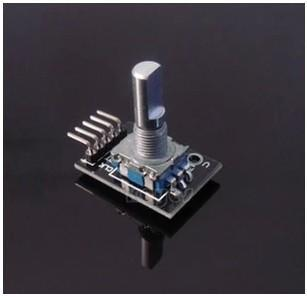• 滿千超商店到店免運費！每次購買都可以累積紅利點數哦！我們有最多Arduino專題製作套件！
•# 360度 旋轉編碼器模組 電子旋轉電位器 旋鈕模組KY-040

NT\$ 50.00
- +

UVW信號增量型編碼器

***************************************************************************

ARDUINO測試程式碼:

int redPin = 2;

int yellowPin = 3;

int greenPin = 4;

int aPin = 6;

int bPin = 7;

int buttonPin = 5;

int state = 0;

int longPeriod = 5000; // Time at green or red

int shortPeriod = 700; // Time period when changing

int targetCount = shortPeriod;

int count = 0;

void setup()

{

pinMode(aPin, INPUT);

pinMode(bPin, INPUT);

pinMode(buttonPin, INPUT);

pinMode(redPin, OUTPUT);

pinMode(yellowPin, OUTPUT);

pinMode(greenPin, OUTPUT);

}

void loop()

{

count++;

{

setLights(HIGH, HIGH, HIGH);

}

else

{

int change = getEncoderTurn();

int newPeriod = longPeriod + (change * 1000);

if (newPeriod >= 1000 && newPeriod <= 10000)

{

longPeriod = newPeriod;

}

if (count > targetCount)

{

setState();

count = 0;

}

}

delay(1);

}

int getEncoderTurn()

{

// return -1, 0, or +1

static int oldA = LOW;

static int oldB = LOW;

int result = 0;

if (newA != oldA || newB != oldB)

{

// something has changed

if (oldA == LOW && newA == HIGH)

{

result = -(oldB * 2 - 1);

}

}

oldA = newA;

oldB = newB;

return result;

}

int setState()

{

if (state == 0)

{

setLights(HIGH, LOW, LOW);

targetCount = longPeriod;

state = 1;

}

else if (state == 1)

{

setLights(HIGH, HIGH, LOW);

targetCount = shortPeriod;

state = 2;

}

else if (state == 2)

{

setLights(LOW, LOW, HIGH);

targetCount = longPeriod;

state = 3;

}

else if (state == 3)

{

setLights(LOW, HIGH, LOW);

targetCount = shortPeriod;

state = 0;

}

}

void setLights(int red, int yellow, int green)

{

digitalWrite(redPin, red);

digitalWrite(yellowPin, yellow);

digitalWrite(greenPin, green);

}